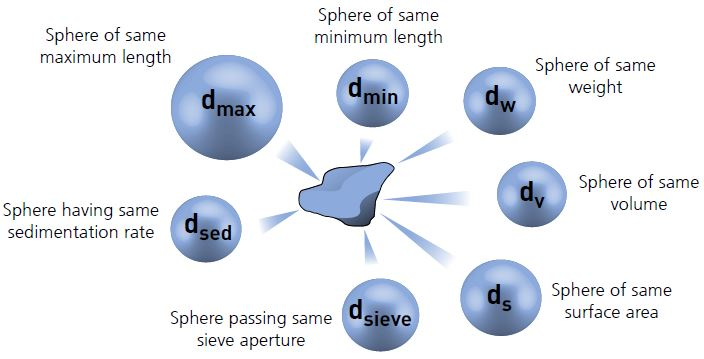# How to define particle size?

Particle size is a very important property in the research field of cementitious materials, e.g. modeling, reaction kinetics.

Particles are 3-dimensional objects, and unless they are perfect spheres, e.g. emulsions or bubbles, they cannot be fully described by a single dimension such as a radius or diameter.

In order to simplify the measurement process, it is often convenient to define the particle size using the concept of equivalent spheres. In this case the particle size is defined by the diameter of an equivalent sphere having the same property as the actual particle such as volume or mass for example.

It is important to realize that different measurement techniques use different equivalent sphere models and therefore will not necessarily give exactly the same result for the particle diameter.

Below is a schematic figure illustrating the definition of particle size based on different equivalent spheres.Definition of particle size